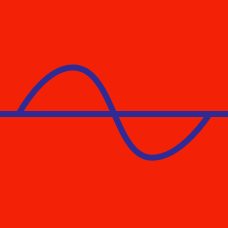Geometry

# Recognizing Trigonometric Graphs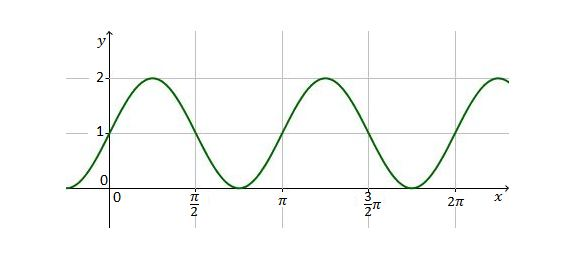The above graph is $y=a \sin bx+c$ with $a > 0$ and $b > 0.$ What is the value of $3a+2b+5c ?$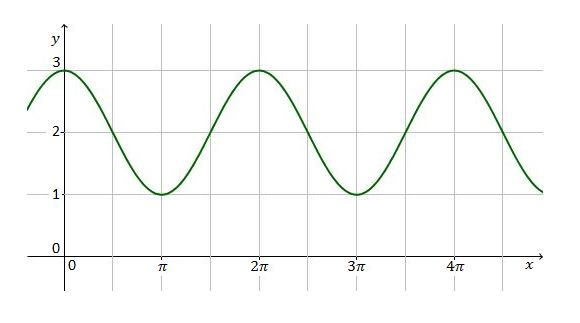Which of the following trigonometric functions corresponds to the above graph?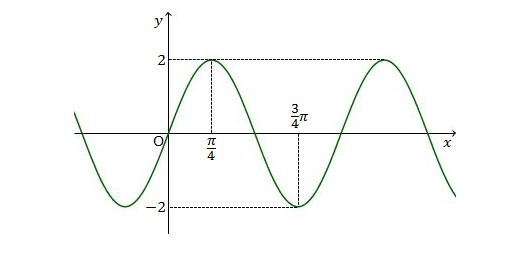The above graph is $y=a \cos (bx+c)$ with $a > 0,$ $b > 0$ and $-\pi < c < \pi.$ What is the value of $4a+3b+6c ?$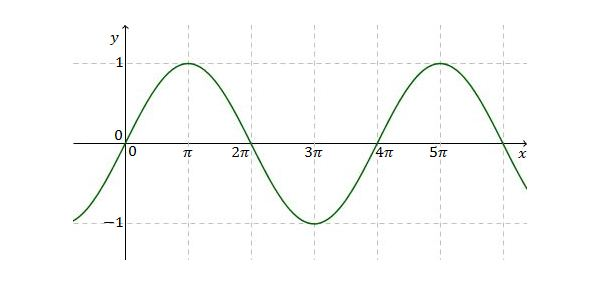Which of the following functions corresponds to the above graph?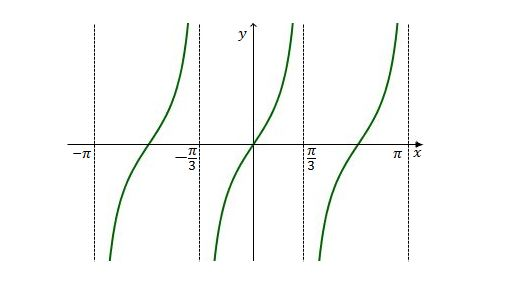If the above is the graph of $y= \tan ax$ with $a > 0,$ what is the value of $16a ?$

×